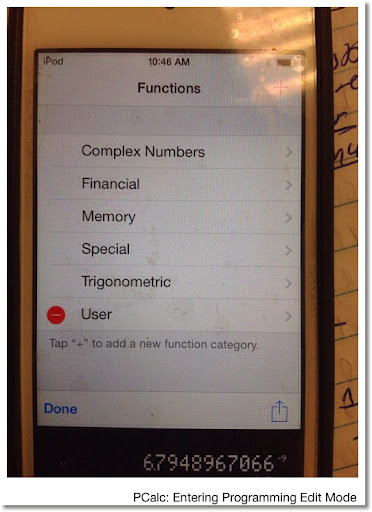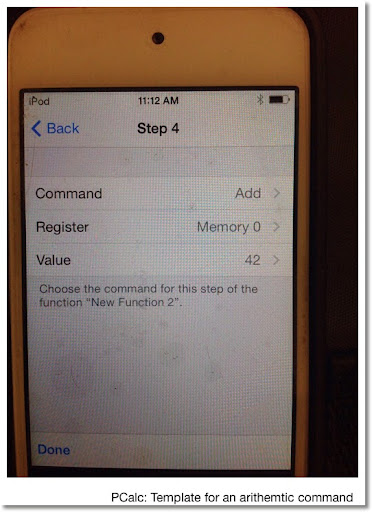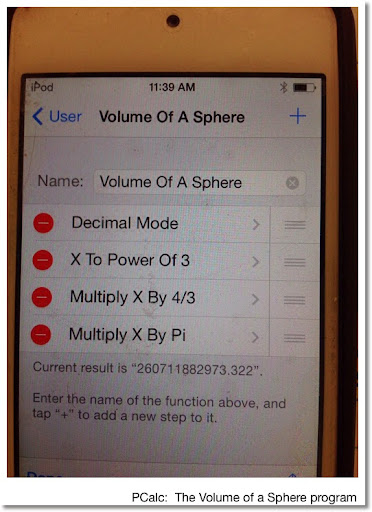## Sunday, May 4, 2014

### PCALC Programming: An Introduction

This blog entry is an introduction to programming the PCalc iOS App by James Thomson. My review of this app can be found here:

http://edspi31415.blogspot.com/2014/04/greetings-from-seattle-and-short-review.html

** Note, PCalc Lite app will not have this functionality. To get it, you have to purchase either the full PCalc iOS app (usually sold for \$9.99), or make an in-app purchase of the appropriate add-on.

Creating or Edit Programs

Starting New Program or Edit a Program:

1. Press the f(x) button.
2. Select Edit (lower left corner of the dialogue box). This will cause a red circle to appear next to "User" and any other customized categories.
3. Select a category (User will work). To edit an existing program, just select it. To create a new program, select the Plus (+) symbol on the upper right hand corner of the screen. You can delete programs by pressing the red circle with a minus sign next to the program name. A red Delete box will appear as an indicator of confirmation.

Programs in PCalc work with registers. The X register is the primary display register. The Y register is the second display register. PCalc also has 10 permanent memory registers (labeled Memory 0 through Memory 9), 16 temporary registers (labeled Register 0 through Register 9, then Register A through Register F), and a tax register.

Everything is done in a sequential manner.

Types of Commands

There are several types of commands.

Mode Commands: they change the mode of PCalc. This includes angle setting (Degrees Mode, Radians Mode) and base setting (Decimal Mode, Octal Mode, Binary Mode, Hexadecimal Mode).

One Argument Commands: A command operates on a register and stores the result in that register. This includes trigonometric and logarithmic commands. This also includes several number operations:

Negate: Multiply the register by -1. This is like the change sign key. (+/-)

Invert: Takes the reciprocal of the value of the designated register. (1/x)

Truncate: Takes the integer part of the value of the register. (INTG/IP)

Exponent: The exponential function (e^x)

Factorial: The factorial function (x!). x must be positive, but does not have to be an integer.

Two Argument Commands: This is the arithmetic operations, power commands, and the Set command. The format is like this:

Command (operation)
Register (result is stored here)
Value (value of a designed register or specified value)

Let A and B be registers, B can also be a value.

Add B To A: A + B is stored in A

Subtract B From A: A - B is stored in A

Multiply A By B: A × B is stored in A

Divide A By B: A ÷ B is stored in A

A To Power of B: A^B is stored in A

(Inverse Power):
A To Power Of 1/B: Bth root of A stored in A (principal root)

Skipping Functions: PCalc allows for Boolean comparisons of values.

Command: Skip (what has to happen for the next commands to be skipped)
Register (register to be compared)
Value (register or value that is compared)
Skip (number of steps if the comparison is true)

For example:
Command: Skip If Greater Than
Register: X
Value: 42
Skip: 1

Skip 1 step if X>42.

There is a plain Skip command to arbitrarily skip a number of commands. This can turn out to be useful. It may take practice to get the Skip commands correct.

There are no loop commands in PCalc (as of this blog post).

You can stop, even invoke the error condition at any time by inserting a Stop or Error command, respectively.

Let's go over a couple of examples.Volume of a Sphere

The volume of a sphere is V = 4/3 * π * r^3, where r is the radius.

This program takes the radius in the X register and calculate the volume of the radius. Please pay careful attention to the order of the program steps.

Volume of a Sphere
Decimal Mode
X To The Power of 3
Multiply X By 4/3
Multiply X By Pi (scroll down the possible list of values to select π).

Test example: The radius of Earth is approximately 3,963 miles. If we treat Earth as a sphere, it's volume would approximately be 260,711,882,973.332 cubic miles.

In more complex programs, I start by making copies of X, Y, and any other required registers into temporary registers. I use temporary registers to store and execute immediate calculations. When all the calculations are finished, I store the results into X, Y, and permanent memory registers (if necessary). The next program, Rect > Polar, will be an example of this.

Convert Rectangular Coordinates to Polar Coordinates

Enter the y coordinate then the x coordinate. The result will have r in the X register and the angle in the Y register. The angle is shown in degrees and ranges from -180° to 180°, similar to most scientific calculators with this function.

Comments are followed by a double backwards slash characters ( \\ ). These are for notes only and are not entered.

Rect > Polar
Decimal Mode
Degrees Mode \\ set PCALC to degrees
Set R0 to X \\ start calculating r = √(x^2 + y^2)
R0 To The Power of 2
Set R1 To Y
R1 To The Power of 2
R0 To The Power of 1/2 \\ Inverse Power command, R0 = r
Set R1 To Y \\ start calculation for angle
Skip 5 If X!=0 \\ skip the next 5 steps if x≠0 - Goto (I)
Skip 2 If Y<0
Set R1 To 90
Skip 10 \\ skip the next 10 steps - Goto (II)
Set R1 To -90
Skip 8 \\ Goto (II)
Divide R1 By X \\ Label (I)
Inverse Tangent R1 \\ atan(R1)
Skip 2 If X>=0 \\ block if x<0 and y≥0
Skip 1 If Y<0
Skip 2 If X>=0 \\ block if x<0 and y<0
Skip 1 If Y>=0
Subtract 180 From R1 \\ R1 = angle
Set X To R0 \\ Label (II) - r is now in register X
Set Y To R1 \\ angle is now in register Y, end of program

How to enter (x,y):
RPN Mode On: y, enter key, x, f(x) key, select Rect > Polar
RPN Mode Off: y, x~y key, x, f(x) key, select Rect > Polar

How to View Results:
RPN Mode On: r is displayed on the X stack, angle on the Y stack
RPN Mode Off: r is displayed, press the x~y key to get the angle

Example data (x,y):
y = 3, x = 3: r = 4.242640687, angle = 45
y = -4, x = 3: r = 5, angle = -53.13010235
y = 2, x = -2: r = 2.82842715, angle = 135
y = -3, x = -2: r = 3.60551275, angle = -123.6900675

My next blog entry will have several more example programs using PCalc.

Eddie

This blog is property of Edward Shore. 2014

### Casio fx-CG50: Sparse Matrix Builder

Casio fx-CG50: Sparse Matrix Builder Introduction The programs can create a sparse matrix, a matrix where most of the entries have zero valu...